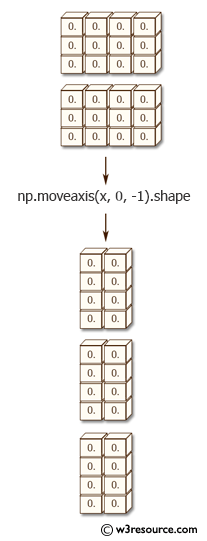﻿ NumPy: Move axes of an array to new positions - w3resource# NumPy: Move axes of an array to new positions

## NumPy: Array Object Exercise-52 with Solution

Write a NumPy program to move axes of an array to new positions. Other axes remain in their original order.

Pictorial Presentation:Sample Solution :-

Python Code :

``````import numpy as np
x = np.zeros((2, 3, 4))
print(np.moveaxis(x, 0, -1).shape)
print(np.moveaxis(x, -1, 0).shape)
```
```

Sample Output:

```(3, 4, 2)
(4, 2, 3)
```

Python Code Editor:

Have another way to solve this solution? Contribute your code (and comments) through Disqus.

What is the difficulty level of this exercise?

Test your Python skills with w3resource's quiz

﻿

## Python: Tips of the Day

Set comprehension:

```>>> m = {x ** 2 for x in range(5)}
>>> m
{0, 1, 4, 9, 16}
```